Practice the AP 9th Class Physical Science Bits with Answers Chapter 4 Atoms and Molecules on a regular basis so that you can attempt exams with utmost confidence.

## AP State Syllabus 9th Class Physical Science Bits 4th Lesson Atoms and Molecules with Answers

Question 1.
When the particles of a substance contain only one type of atoms, that substance is called a / an
A) molecule
B) element
C) compound
D) atom
B) element

Question 2.
Combination of atoms of same elements is known as
A) molecule
B) element
C) compound
D) atom
A) moleculeQuestion 3.
If atoms of different elements combine then ………………. is formed.
A) molecule
B) element
C) compound
D) atom
C) compound

Question 4.
Symbol of potassium
A) Pb
B) Na
C) Fe
D) K
D) K

Question 5.
The other name of tungsten
A) Natrium
B) Kalium
C) Wolfram
D) Cuprum
C) Wolfram

Question 6.
Correct method of representing a symbol
A) BE
B) he
C) al
D) Cr
D) Cr

Question 7.
Which of the following is octatomic ?
A) nitrogen
B) oxygen
C) carbon
D) sulphur
D) sulphur

Question 8.
The valency of sulphate is
A) 2 –
B) 2 +
C) 3 –
D) 3 +
A) 2 –

Question 9.
In NH4Cl, the cation is
A) Cl
B) NH4
C) NH4Cl
D) None
B) NH4Question 10.
Chemical formula of aluminium sulphate is
A) Al2SO4
B) (Al2)2(SO4)3
C) Al2(SO4)3
D) AlSO4
C) Al2(SO4)3

Question 11.
Molecular mass of H2SO4 is
A) 98 u
B) 89 u
C) 49 u
D) 106 u
A) 98 u

Question 12.
Molar mass of 1.5055 × 1023 number of calcium atoms
A) 20 gm
B) 40 gm
C) 10 gm
D) 30 gm
C) 10 gm

Question 13.
Convert into moles : 8 gm of Mg.
A) 0.3
B) 3
C) 2
D) 0.2
A) 0.3

Question 14.
Among the following which element has more number of atoms ?
A) Sulphur
B) Calcium
C) Nitrogen
D) Carbon
D) Carbon

Question 15.
“Mass is neither created nor destroyed during a chemical reaction”. This is
known as ………………..
A) Law of constant proportions
B) Law of multiple proportions
C) Law of conservation of mass
D) Law of conservation of energy
C) Law of conservation of mass

Question 16.
Dalton proposed atomic theory based on ………………………
A) Law of conservation of mass
B) Law of constant proportions
C) Both A & B
D) Neither A nor B
C) Both A & BQuestion 17.
According to Dalton atom is ………………….. particle.
A) indivisible
B) tiny
C) both A & B
D) none of these
C) both A & B

Question 18.
The word (atom) is derived from a Greek word “atomio” means ………………….
A) indivisible
B) divisible
C) both A & B
D) none of these
A) indivisible

Question 19.
……………….. are the building blocks of all matter.
A) Atoms
B) Molecules
C) Elements
D) Compounds
A) Atoms

Question 20.
Latin word for water is………………..
A) hydro
B) oxy
C) helios
D) none of these
A) hydro

Question 21.
Latin word for acid is ………………….
A) hydro
B) oxy
C) helios
D) atomio
B) oxy

Question 22.
Symbol of Beryllium is …………………
A) Ba
B) Be
C) Br
D) B
B) Be

Question 23.
Symbol for nitrogen is ………………….
A) Ni
B) Na
C) N
D) No
C) NQuestion 24.
Symbol for gold …………………
A) G
B) Ga
C) Ge
D) Au
D) Au

Question 25.
Cl2 is the formula for ………………………..
A) Chlorine
C) Chromium
D) Calcium
A) Chlorine

Question 26.
We are protected by shielding the earth from some harmful rays of the sun by ……………….
A) atmosphere
B) ozone layer
C) clouds
D) sky
B) ozone layer

Question 27.
The number of atoms constituting a molecule is known as its …………………….
A) valency
B) atomicity
C) atomic number
D) mass number
B) atomicity

Question 28.
The atomicity of sodium is ……………….
A) 1
B) 2
C) 3
D) 4
A) 1

Question 29.
The combining capacity of one atom with another atom is its ……………………
A) valency
B) atomicity
C) atomic number
D) mass number
A) valency

Question 30.
Valency of Argon is ……………………
A) 0
B) 1
C) 2
D) 3
A) 0Question 31.
Valency of Carbon is ……………………
A) 1
B) 2
C) 3
D) 4
D) 4

Question 32.
A positively charged ion is called …………………
B) anion
C) cation
D) complex ion
C) cation

Question 33.
A negatively charged ion is called …………………
B) anion
C) cation
D) multiple ion
B) anion

Question 34.
In NH4OH the anion is ………………..
A) OH
B) NH4+
C) NH3+
D) NH+
A) OH

Question 35.
Standard reference for measuring atomic masses of other elements is
A) atomic mass of carbon – 12
B) atomic mass of carbon – 14
C) atomic mass of oxygen – 16
D) atomic mass of oxygen – 18
A) atomic mass of carbon – 12

Question 36.
The number of times one atom of given element is heavier than 1/12th part of atomic mass of carbon – 12 is called as ……………..
A) valency
B) atomicity
C) atomic mass
D) atomic number
C) atomic mass

Question 37.
Atomic mass of Magnesium is …………………..
A) 8
B) 10
C) 12
D) 24
D) 24Question 38.
Formula of silver nitrate …………………
A) AgNO2
B) AgSO4
C) AgNO3
D) Ag(NO3)2
C) AgNO3

Question 39.
Molecular mass of sodium carbonate is ………………… U.
A) 108
B) 104
C) 110
D) 106
D) 106

Question 40.
A) 6.022 × 1020
B) 6.022 × 1021
C) 6.022 × 1022
D) 6.022 × 1023
D) 6.022 × 1023

Question 41.
Molar mass of water = ………………….. U.
A) 16
B) 18
C) 20
D) 22
B) 18

Question 42.
Number of particles present in 32 g of oxygen molecule are ……………………
A) 6.022 × 1020
B) 3.011 × 1023
C) 6.022 × 1022
D) 6.022 × 1023
D) 6.022 × 1023

Question 43.
Number of moles of 22g of carbon dioxide is ………………….
A) 1
B) 0.25
C) 0.75
D) 0.5
D) 0.5

Question 44.
The valency of Copper in Cu2O is …………………
A) + 1
B) + 2
C) + 3
D) – 1
A) + 1

Question 45.
Number of particles in 7.75 g of phosphorous is …………………
A) 6.022 × 1023
B) 3.011 × 1023
C) 1.5055 × 1023
D) 6.022 × 1022
C) 1.5055 × 1023Question 46.
Symbol of nitrate is ………………
A) NO3
B) NO2
C) N3-
D) N
A) NO3

Question 47.
Statement – I : The symbol of chloride Ion is Cl.
Statement – II : The symbol of ammonium ion is 4+.
A) Both the statements are true.
B) Statement – I is true and statement – II is false.
C) Statement -I is false and statement – II is true.
D) Both the statements are false.
A) Both the statements are true.

Question 48.
Symbol of sodium ion is ………………..
A) Na
B) Na2+
C) Na3+
D) Na+
D) Na+

Question 49.
Match the following.

 a) Calcium nitrate i) HNO3 b) Nitric acid ii) (NH4)3PO4 c) Ammonium chloride iii) Ca(NO3)2 d) Ammonium phosphate iv) NH4Cl

A) a → iii, b→ i, c → iv, d → ii
B) a → i, b → ii, c → iii, d → iv
C) a → ii, b → iii, c → iv, d → i
D) a → iv, b → i, c → ii, d → iii
A) a → iii, b→ i, c → iv, d → ii

Question 50.
The formula of Aluminium Carbonate is ……………………
A) AlCO3
B) Al2CO3
C) Al2(CO3 )3
D) Al(CO3)2
C) Al2(CO3 )3

Question 51.
The symbol of Zinc ion is ……………….
A) Zn
B) Zn+
C) Zn2+
D) Zn3+
C) Zn2+

Question 52.
Which of the following statement is not given by Dalton ?
Statement (A) : If mass was to be conserved, then all elements must be made up of extremely small particles.
Statement (B) : If law of constant proportion is to be followed, the particles of same substance be either same or different.
A) A only
B) B only
C) both A & B
D) none
B) B onlyQuestion 53.
Who is correct ?
Srinu : Elements are made up of atoms.
Mohan : Atoms are made up of elements.
A) Srinu
B) Mohan
C) Both
D) Neither Srinu nor Mohan
A) Srinu

Question 54.
Assertion (A) : The atomic mass of magnesium is 24.
Reason (R) : Magnesium atom is 24 times heavier than that of 1 /12 of mass of carbon.
A) A and R are true, A is supported by R
B) A and R are true, A is not supported by R
C) A and R are false
D) A is true but R is false
A) A and R are true, A is supported by R

Question 55.
Assertion (A) : Atomic mass has no units.
Reason (R) : Atomic mass is an expression of ratio.
A) A and R are true, A is supported by R
B) A and R are true, A is not supported by R
C) A and R are false
D) A is true but R is false
A) A and R are true, A is supported by R

Question 56.
An iron rod changed into iron oxide by rusting. Guess how the weights before and after rusting of the iron rod are changed,
if a = weight of the iron rod
b = weight of the iron oxide
A) a > b
B) b > a
C) a = b
D) we cannot say
C) a = b

Question 57.
In the experiment of “To prove law of conservation of mass”, Manoj found that weight of ‘Mg’ in a closed test tube is not equal to the weight of ‘MgO’ after burning.
The reason may be
A) ‘Law of conservation of mass’ is not applicable to some chemical changes.
B) Some gas was escaped in this experiment.
C) He was used simple balance to weight.
D) Above all
B) Some gas was escaped in this experiment.

Question 58.
CO : 1 : 1 :: CO2 : ………………… ?
A) 1 : 1
B) 2 : 1
C) 1 : 2
D) 2 : 3
C) 1 : 2

Question 59.
Molecules in water is H2O, then molecules in the Hydrogen is
A) H
B) H2
C) A or B
D) No molecules
B) H2Question 60.
Why did symbol of copper taken as ‘Cu’ ?
A) Latin name of copper is cuprum.
B) Original spelling of copper is cupper.
C) Symbols of all metals should be taken in two letters.
D) Above all
A) Latin name of copper is cuprum.

Question 61.
Statement (A) : Symbol of carbon is ‘C’ whereas symbol of calcium is ‘Ca’.
Reason (R) : Eventhough carbon and calcium have ‘C’ as its initial, carbon occurs first in periodic table. So, its symbol was taken as ‘C’ and calcium symbol was taken as ‘Ca’.
A) Both A and R are true
B) Both A and R are false
C) A is true but R is false
D) A is false but R is true
A) Both A and R are true

Question 62.
Generally all inert gases like He, Ne, Ar, Kr, Xe are available as monoatomic elements.
Guess the reason.
A) They are more reactive
B) Their valency is zero
C) Their valency is less than 8
D) They are unstable
B) Their valency is zero

Question 63.
If ‘x-1‘ and Na’x’, then ‘x’ may be
A) carbon
B) cromium
C) chlorine
D) copper
C) chlorine

Question 64.
The valencies of ‘Mg’ and ‘O’ are ………………… respectively in MgO.
A) 1, 1
B) 2, 2
C) 1, 2
D) 2, 1
B) 2, 2

Question 65.
‘X2 Y’, ‘X’ H ‘Y’, ‘X’ OH
Guess X and Y.
A) X = Na ; Y = OH
B) X = Na ; Y = CO3
C) X = CO3 ; Y = Zn
D) X = Zn ; Y = CO3
B) X = Na ; Y = CO3

Question 66.
By using Ag+ Cl, Na+, OH
How many substances can be formed?
A) 2
B) 3
C) 4
D) 6
C) 4

Question 67.
Molar mass of molecule x = 2 gr
Molar mass of molecule y = 32 gr
Molar mass of molecule x2 y = 18 gr Predict x and y.
A) x = H2 ; y = O2
B) x = O2 ; y = H2
C) x = H2; y = Cl2
D) x = Cl2 ; y = H2
A) x = H2 ; y = O2Question 68.
No. of molecules in 44 grams of CO2 is equal to
A) no. of molecules in 18 gr of H2O
B) no. of molecules in 2 grams of H2
C) no. of molecules in 32 grams of O2
D) any one of the above
D) any one of the above

Question 69.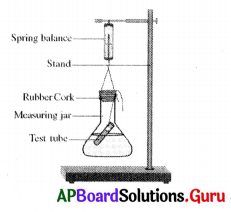To prove ‘conservation of the mass’, what should be weight ?
B) weight of potassium iodide
C) weight of lead iodide and potassium nitrate
D) above all
D) above all

Question 70.
In the above given experiment, this precaution must be taken
A) put the cork tightly
B) measure components accurately
C) while measuring leave the apparatus freely
D) above all
D) above all

Question 71.
Law of conservation of mass was proposed by
A) Antoine Lavoisier
B) Joseph L. Proust
C) John Dalton
D) Landolt
A) Antoine Lavoisier

Question 72.
Law of conservation of mass was experimentally verified by
A) Antoine Lavoisier
B) Joseph L. Proust
C) John Dalton
D) Landolt
D) Landolt

Question 73.
Law of constant proportions was proposed by
A) Antoine Lavoisier
B) Joseph L. Proust
C) John Dalton
D) Landolt
B) Joseph L. Proust

Question 74.
What is the original name of Kanada, an Indian sage, who proposed anu and paraman I ?
A) Vaishesika Sutra
B) Rishi
C) Kasyapa
C) KasyapaQuestion 75.
Initial letter of an element written in capitals should represent that a particular element. This was proposed by
A) John Dalton
B) Landolt
C) John Berzelius
D) Wilhelm Ostwald
C) John Berzelius

Question 76.
The word ‘mole’ was first introduced by
A) John Berzelius
B) Wilhelm Ostwald
C) John Dalton
B) Wilhelm Ostwald

Question 77.
Number of particles present in 9 grams of aluminium
A) 2.007 × 1023
B) 3.011 × 1023
C) 18.066 × 1023
D) 6.022 × 1023
A) 2.007 × 1023

Question 78.
Father of modern chemistry is ………………….
A) Levoisier
B) Proust
C) Dalton
D) Landolt
A) Levoisier

Question 79.
Greek name of sun is ………………
A) hydro
B) oxy
C) helios
D) atomio
C) helios

Question 80.
The latin name of Mercury is
A) Aurum
B) Cupsum
C) Kalium
D) Hydragyrum
D) Hydragyrum

Question 81.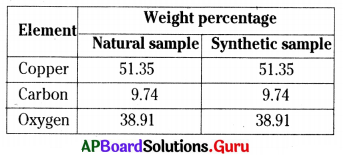From the table particularly we can prove that
A) Law of conservation of mass
B) Law of constant proportions
C) Law of conservation of energy
D) Above all
B) Law of constant proportionsQuestion 82.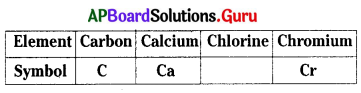The missing symbol is
A) Ch
B) Ce
C) Cl
D) Chl
C) Cl

Question 83.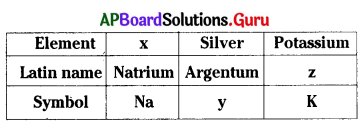x, y, z are ………………, ………………., …………….. respectively.
A) Sodium, Ag, Kalium
B) Kalium, Sodium, Ag
C) Ag, Sodium, Kalium
D) Ag, Kalium, Sodium
A) Sodium, Ag, Kalium

Question 84.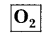From the given substance, select the wrong statement.
A) It is a molecule of oxygen
B) It has two elements
C) It contains two oxygen atoms
D) It is not a compound
B) It has two elements

Question 85.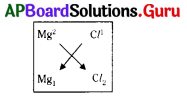The formula of magnesium chloride is
A) MgCl2
B) Mg2Cl
C) MgCl
D) Mg2Cl2
A) MgCl2

Question 86.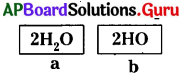From the given data single molecule of water is
A) ‘a’
B) ‘b’
C) ‘a’ and ‘b’
D) neither ‘a’ nor ‘b’
D) neither ‘a’ nor ‘b’

Question 87.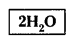By using this, select the wrong statement.
A) The atomicity of water molecule is 6.
B) Water molecule contains 3 atoms.
C) The above indicates 2 molecules of water.
D) It is not exists because of unstable.
A) The atomicity of water molecule is 6.Question 88.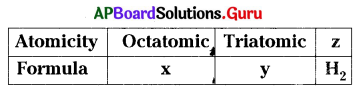x, y, z are ………………., ………………, ……………….. respectively.
A) O8, S3, diatomic
B) S8, C3, monoatomic
C) S8, O3, diatomic
D) S8, O3, diatomic
C) S8, O3, diatomic

Question 89.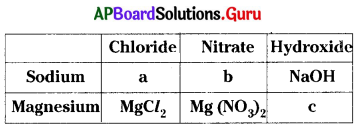a, b, c are ……………., ………………., ……………… (respectively)
A) NaCl, Na2NO3, MgOH
B) NaCl2, NaNO3, Mg(OH)2
C) NaCl, NaNO3, MgOH
D) NaCl, NaNO3, Mg(OH)2
D) NaCl, NaNO3, Mg(OH)2

Question 90.
Find the odd one according to their net charge of ion.
A) Hydrogen, sodium, potassium
B) Magnesium, calcium, zinc
C) Aluminium, iron, silver
D) Ammonium, copper, silver
C) Aluminium, iron, silver

Question 91.
Antoine Lavoisier is appreciable because,
A) He proposed the law of conservation of mass.
B) He is the father of modern chemistry.
C) He proposed the law of constant proportions.
D) A and B
D) A and B

Question 92.
Atomic mass can be measured accurately by using this device.
A) Mass spectrometer
B) Light spectrometer
C) Electronic balance
D) None
A) Mass spectrometer

Question 93.
No. of atoms in 16 g of oxygen.
A) 6.022 × 1023
B) 3.011 × 1023
C) 12.044 × 1023
D) None
A) 6.022 × 1023

Question 94.
In a soda water there is 44 grams of CO2 is mixed with 18 grams of water. The no. of molecules of H2CO3 (soda water) is
A) 6.022 × 1023
B) 3.011 × 1023
C) 12.044 × 1023
D) None
A) 6.022 × 1023Question 95.
Avogadro is appreciable, because he found that one mole of any substance contains …………….. molecules.
A) 6.2 × 1022
B) 6.4 × 1019
C) 6.02 × 1023
D) Countless
C) 6.02 × 1023

Question 96.
The great mole concept was introduced by ……………….. and he is appreciable.
B) Ostwald
C) Dalton
D) Lavoisier
B) Ostwald

Question 97.
‘Washing soda’ is the common name of
A) Na2CO3
B) NaHCO3
C) Na2SO4
D) Na3PO4
A) Na2CO3

Question 98.
Match the following.

 a) Copper i) Aurum b) Potassium ii) Cuprum c) Gold iii) Kalium d) Silver iv) Argentum

A) a → iii, b → iv, c → i, d → ii
B) a → ii, b → iii, c → i, d → iv
C) a → i, b → ii, c → iii, d → iv
D) a → iv, b → i, c → ii, d → iii
B) a → ii, b → iii, c → i, d → iv

Question 99.
Based on atomicity match Set – A with Set – B :

 Set – A Set – B P. Monoatomic x. Fluorine Q. Diatomic y. Helium R. Triatomic z. Ozone

A) P – y, Q – x, R – z
B) P – y, Q – z, R – x
C) P – z, Q – y, R – x
D) P – z, Q – x, R – y
A) P – y, Q – x, R – z

Question 100.
The correct order in the activity to observe law of conservation of mass is
P. Take 100 ml of silver nitrate solution in a chemical flask and 5 ml of sodium chloride in a test tube.
Q. Due to reaction between these two white precipitate will form.
R. Add sodium chloride solution to silver nitrate solution.
S. Weight the two and record the sum of their weights.
T. After reaction weigh it again and it will be equal to the previous reading.
A) P, R, S, Q, T
B) P, S, R, Q, T
C) P, S, Q, R, T
D) P, S, R, T, Q
A) P, R, S, Q, T

Question 101.
Uma : Mass is neither created nor destroyed during a chemical reaction. Rama : Matter is conserved in a chemical reaction.
A) Uma is correct, Rama is incorrect.
B) Bot Uma and Rama are correct.
C) Both Uma and Rama are incorrect.
D) Uma is incorrect, Rama is correct.
A) Uma is correct, Rama is incorrect.Question 102.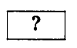: Sodium carbonate : : Al2(SO4)3 : Aluminium sulphate
A) NaCO3
B) Na2(CO3)2
C) Na2(CO3)2
D) Na2CO3
D) Na2CO3

Question 103.
David : By transfer of electrons atoms – can attain octet
Dravid : By sharing of electrons atoms – can attain octet
A) David incorrect, Dravid correct.
B) Both David and Dravid are incorrect.
C) Both David and Dravid are correct.
D) David correct, Dravid incorrect.
C) Both David and Dravid are correct.

Question 104.
A given chemical substance always contains the same elements combined in a fixed proportion by mass. Guess the compounds formed in the ratio of 1 : 1 and 1 : 2 by Carbon and Oxygen.
A) CO2, CO
B) CO, CO3
C) CO2, CO3
D) CO, CO2
D) CO, CO2

Question 105.
A neutral metal atom becomes dipositive ion by loosing two”electrons. In the same way a neutral non-metal atom becomes uni-negative ion by gaining one electron. The formula of a compound which is formed by these two ions is
A) m2
B) m2x1
C) m2x3
D) m2x2
D) m2x2

Question 106.
We can test the law of conservation of mass by the reaction between barium chloride and sodium sulphate. While doing the experiment, one of the precautions you have taken is
A) Reactants should be taken in solid state.
B) Cork was not fitted while the reaction is taking place.
C) Common balance is used to weigh the substances.
D) Flask was tilted and swirled for the mixing of two solutions.
D) Flask was tilted and swirled for the mixing of two solutions.

Question 107.
The apparatus which is not required in the experiment mentioned in question number (8) is
A) Test tube
B) Beaker
C) Stand
B) Beaker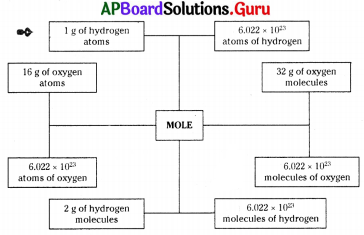Observe the following flow chart and answer 10 & 11 questions.
Question 108.
Based on the above flow chart, generalised statement is
P : In any substance the no. of particles in one mole is constant.
Q : Irrespective of quantity of a matter, the no. of atoms or molecules in it is constant.
R : There is no relation between the mole and the substance.
A) Q only
B) P and Q
C) R only
D) P only
A) Q onlyQuestion 109.
hi the above flow chart, the number 6.022 × 1023 is
B) Mass number (A)
C) Atomic number (Z)
D) Atomic mass unit (amu)

Question 110.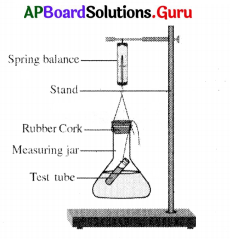Wrongly labelled part in the above diagram is
A) Test tube
B) Measuring jar
C) Rubber cork
D) Spring balance
A) Test tube

Question 111.
The molecular mass of a substance is the sum of the atomic masses of all the atoms in a molecule of the substance. If the atomic masses of hydrogen, sulphur and oxygen are 1, 32, 16 successively then the molecular mass of sulphuric acid is
A) 98 U
B) 49 U
C) 97 U
D) 89 U
A) 98 U

Question 112.
Some symbols of elements are based on their English names. Some other are based on their Latin names. Which of the following elements have symbols based on Latin names ?
i) Sodium
ii) Neon
iii) Gold
iv) Argon
A) (i) and (iii)
B) (i) and (iv)
C) (ii) and (iv)
D) (i) and (ii)
A) (i) and (iii)

Question 113.
No. of molecules present in 18 grams of H2O
A) 6.02 × 1022
B) 6.02 × 1023
C) 6.02 × 1032
D) 6.02 × 1035
B) 6.02 × 1023

Question 114.
If valency of Mg is ‘+2’ and valency of Sulphate (SO4) is ‘-2’, then the formula of compound formed by these
A) Mg2SO4
B) Mg (SO4)2
C) MgSO4
D) Mg3(SO4)2
A) Mg2SO4

Question 115.
Homogeneous molecule from the following
A) H2O
B) N2
C) N2O3
D) FeSO4
B) N2Question 116.
How many moles are contained in 180 grams of water ?
A) 100
B) 10
C) 180
D) 18
B) 10

Question 117.
What is the valency of silver ?
A) 1
B) 2
C) 3
D) 4
A) 1

Question 118.
The Common name of Hydrogen Monoxide is
A) Water
B) Salt
C) Washing Soda
D) Baking Soda
A) Water

Question 119.
Valencies of nitrogen and hydrogen are 3 and 1 respectively. The formula of ammonia molecule formed by these
two atoms
A) NH3
B) NH4
C) N3H
D) N4H
A) NH3

Question 120.
P : The atmoicity of Oxygen is 3
Q : The formula of ozone is O3.
A) P – correct, Q – incorrect
B) P – incorrect, Q – correct
C) Both P & Q incorrect
D) Both P & Q correct
D) Both P & Q correct

Question 121.
One of the precautions to be taken in the conduction of experiment on law of conservation of mass is
A) Test tube should not be tilted
B) Test tube should be tilted
C) Test tube should be immersed in the conical flask
D) Test tube should be kept outside the conical flask
B) Test tube should be tilted

Question 122.
Ornaments that we wear contains ………………… metal
A) Mercury
B) Sodium
C) Calcium
D) Gold
D) GoldQuestion 123.
The latin name of the element Tungsten is
A) Aurum
B) Plumbum
C) Kalium
D) Wolfram
D) Wolfram

Question 124.
Molecular formula of Ozone is ………………….
A) O3
B) O2
C) O
D) O8